# NCERT Solutions class 12 Economics National income accounting## myCBSEguide App

CBSE, NCERT, JEE Main, NEET-UG, NDA, Exam Papers, Question Bank, NCERT Solutions, Exemplars, Revision Notes, Free Videos, MCQ Tests & more.### Microeconomics

• Introduction
• Theory o Consumer Behavior
• Production and Costs
• Theory of Firm Under Perfect Competition
• Market Competition
• Non Competitive Markets

### Macroeconomics

• Introduction
• National Income Accounting
• Money and Banking
• Income Determination
• Government Budget and Economy
• Open Economy Macroeconomics

## CHAPTER TwoNational Income Accounting

1. Some Basic Concepts of Macroeconomics
2. Circular Flow of Income and Methods of Calculating National Income
1. The Product or Value Added Method
2. Expenditure Method
3. Income Method
3. Some Macroeconomic Identities
4. Goods and Prices
5. GDP and Welfare

## NCERT Solutions class 12 Economics National income accounting

1. What are the four factors of production and what are the remunerations to each of these called?

Ans: The four factors of production are:

i. Land- It denotes the natural resources like air, water, soil, etc. The payment that is paid by the firms to acquire these services is called rent.

ii. Labour- It refers to the physical and mental effort required to do a work. For example, engineer, manager, worker, etc. The payment made to the labour in exchange of his/her services is called as wage.

iii. Capital- It refers to the monetary investments and physical and tangible investments like machinery, buildings, technology, tools, etc, which assists in production process. The payment received in exchange of these services is called interest.

iv. Entrepreneur- It refers to the individual who undertakes the risk to organise the production process. Entrepreneurs are the risk takers and often are the innovators of new techniques. They receive profit in exchange of their entrepreneurship.

The remunerations paid to the factors of productions are called factor payments or factor incomes. These are the aggregation of rent, wage, interest and profit.

### 2.Why should the aggregate final expenditure of an economy be equal to the aggregate factor payments? Explain.

Ans: In a two sector economy, consisting of households and firms, the only way in which the households can dispose their income is on the goods and services produced by the firms. The factors of production use their remuneration to purchase goods and services. Thus, the income will come back to the producers in the form of sales’ revenue. So, there is no difference between the amount that firms distribute in the form of factor payments and consumption expenditure incurred by the households. The same process continues year after year. However, if there has been any leakage in the form of savings, imports or taxes, then there arises a difference between the aggregate consumption expenditure and aggregate factor payments.

In the case of some leakage, the households will spend less than their factor incomes. Consequently, the firms will receive lesser amount in the form of revenue, which will reduce the production level and employment level. This process will continue in every successive round and production and employment levels will continue to drop. Thus, the equality between the aggregate consumption expenditure and the aggregate factor payments is very necessary for the smooth functioning of the economy.

### 3.Distinguish between stock and flow. Between net investment and capital, which is a stock and which is a flow? Compare net investment and capital with flow of water into a tank.

Ans:

 Stock Flow 1. The variables that are measured at a particular point of time. For example, bank balance as on 1st Oct 2010 is Rs.5000. 1. The variables that are measured over an interval of time. For example, interest earned on bank deposits for 1 year, i.e. from 1 Oct-2009 to 30 Sep 2010. 2. It has no time dimensions. 2. It has time dimensions, like 1 year, 6 months, 10 days, etc. 3. Examples: Capital, bank deposits, water in a tank. 3. Examples: Capital formation, interest on capital, water flowing in a stream.

An example of stock can be the amount or level of water in a tank. At any point of time the amount or the level of water in a tank can be measured. Similarly, the capital is also a stock variable, as the capital can also be measured at any point of time.

Now, if water is flowing out of a tank through a tap, then the level of water will change over time. The difference in water level over an interval of time is an example of a flow variable. Similarly, net investment gives the difference in the investment level over a period of time.

### 4. What is the difference between planned and unplanned inventory accumulation? Write down the relation between change in inventories and value added of a firm.

Ans: The stock of unsold goods (finished and semi-finished), which a firm carry forward from one year to another year is termed as an inventory.

Inventory accumulation can be planned or unplanned. The planned inventory accumulation refers to the inventory that a firm can anticipate or plan. For example, a firm want to raise its inventory from 1000 to 2000 units of denims and expects sales to be 10000 units. Thereby, it produces 10000 + 1000 units, i.e. 11000 units (in order to raise the inventory by 1000 units). If, at the end of the year it is found that the actual sales that got realised were also 10000, then the firm experiences the rise in its inventory from 1000 to 2000 units. The closing balance of inventory is calculated in the following manner:

Final Inventory = Opening inventory + Production – Sale

= 1000 + 11000 – 10000

= 2000 units of denims

#### In this case the inventory accumulation is equal to the expected accumulation. Hence, this is an example of a planned inventory accumulation.

Unplanned inventory accumulation is an unexpected change in an inventory. There is an unplanned accumulation in an inventory when the actual sales are unexpectedly low or high. For example, let us assume, a firm want to raise inventory from Rs 1000 to 2000 and expects sales to be 10000 and thereby produces 11000 units of denims. If, at the end of the year, the actual sales realised were 9000 units only, which were not anticipated by the firm and therefore the inventory rose by 3000 units. The unexpected inventory accumulation is calculated as:

Final Inventory = Opening inventory + Production – Sale

= 1000 + 11000 – 9000

= 3000 units of denims

Hence, this is example of unexpected inventory accumulation.

The relation between value added and the change in inventory is shown by the given equation:

Gross value added by a firm = Sales + Change in inventory – Value of intermediate goods

It implies that, as inventory increases, the value added by a firm will also increase, thus confirming the positive relationship between the two.

### 5.Write down the three identities of calculating the GDP of a country by the three methods. Also briefly explain why each of these should give us the same value of GDP.

Ans: GDP can be calculated by the following three methods:

(a) Income method

GDP = Total payments made to the factors of production

GDP =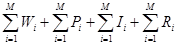…………………………. (i)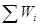represents total wages and salaries received by i = th households.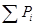represents total profit received by i-th households.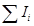represents total Income received by i-th households.represents total Rent received by i-th households.

Equation (1) can be simplified as

GDP = W + R + I + P

(b) Value added or product method

GDP = Sum of gross value added by all firms in an economy

or GDP =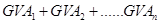Where,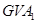represents gross value added by the 1st firm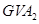represents gross value added by the 2nd firm and so on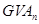represents gross value added by the nth firm

Therefore,

GDP =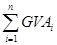(c) Expenditure method or final consumption method

GDP = Sum total of revenues that firms earn

Or

##### GDP = Total consumption + Investment + Government Consumption expenditure + Net exports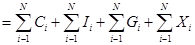As households spend some part of their income on imports, some portion of consumption expenditure also comprises of imports, which are denoted by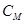. Similarly, some part of the investment expenditure and government consumption expenditure is spent on the foreign investment goods and imports. These portions of investment and government consumption expenditure are denoted by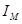and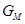respectively. Thus, the final households’ consumption expenditure, investment expenditure and final government expenditure that are spent on the domestic firms are denoted by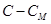,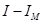and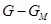respectively.

Substituting these values in the above equation

##### GDP = C –+ I –+ G –+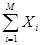= C + I + G +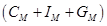= C + I + G + X – M

The three methods give the same result for measuring GDP because what is produced in the economy is either consumed or invested. The three methods depict the same picture of an economy from three different angles. While the product method presents the value added or total production, the income method depicts the income earned by all the factors, lastly, the expenditure method presents the expenditure incurred by all the factors. In the economy, the producer employs four factors of production to produce final goods and earns revenue by sale, which is equivalent to the total value addition by the firm. The firms pay remunerations to the factors, which act as the income of all the factors.

These remunerations are equivalent to the factors’ contributions to the value addition. These factor incomes are then expended on the goods and services, which verifies the equality between the factor income and expenditure. Hence, the three methods will always give the same value of GDP.

### 6.Define budget deficit and trade deficit. The excess of private investment over saving of a country in a particular year was Rs 2,000 crores. The amount of budget deficit was (-) Rs 1,500 crores. What was the volume of trade deficit of the country?

Ans: Budget Deficit

The excess of government expenditure over government income is termed as budget deficit.

Budget Deficit = G – T

Where,

‘G’ represents government expenditure

‘T’ represents government income

Trade deficit measures the excess of import expenditure over the export revenue of a country.

Trade Deficit = M – X

Where,

‘M’ represents expenditure on imports

‘X’ represents revenue earned by exports

It is given that,

I – S = Rs.2000 crores.

G – T = (-) Rs.1500 crores.

Therefore,

Trade deficit = [I – S] + [G – T]

= 2000 + [-1500]

= Rs.500 crores.

### 7. Suppose the GDP at market price of a country in a particular year was Rs 1,100 crores. Net Factor Income from Abroad was Rs 100 crores. The value of Indirect taxes – Subsidies was Rs 150 crores and National Income was Rs 850 crores. Calculate the aggregate value of depreciation.

Ans: National Income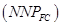= Rs.850 crores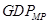= Rs.1100 crores

Net factor income from abroad = Rs.100 crores

Net indirect taxes = Rs.150 crores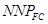=+ Net factor income from abroad – Depreciation – Net indirect taxes

Putting these values in the formula,

850 = 1100 + 100 – Depreciation – 150

850 = 1100 – 50 – Depreciation

850 = 1050 – Depreciation

Depreciation = 1050 – 850 = Rs.200 crores

So, depreciation is Rs.200 crores.

### 8. Net National Product at Factor Cost of a particular country in a year is Rs 1,900 crores. There are no interest payments made by the households to the firms/government, or by the firms/government to the households. The Personal Disposable Income of the households is Rs 1,200 crores. The personal income taxes paid by them is Rs 600 crores and the value of retained earnings of the firms and government is valued at Rs 200 crores. What is the value of transfer payments made by the government and firms to the households?

Ans:= Rs.1900 crores

PDI = Rs.1200 crores

Personal income tax = Rs.600 crores

Value of retained earnings = Rs.200 crores

PDI =– Value of retained earnings of firms and government + value of transfer payments – personal tax

1200 = 1900 – 200 + Value of transfer payments – 600

1200 = 1100 + Value of transfer payments

Value of transfer payment = 1200 – 1100 = Rs 100 crores

### 9. From the following data, calculate Personal Income and Personal Disposable Income.

 Rs (Crore) (a) Net Domestic Product at factor cost 8,000 (b) Net Factor Income from abroad 200 (C) Undisbursed Profit 1,000 (d) Corporate Tax 500 (e) Interest Received by Households 1,500 (f) Interest Paid by Households 1,200 (g) Transfer Income 300 (h) Personal Tax 500

Ans: Personal Income =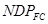+ Net factor income from abroad (NFIA) + Transfer Income – Undistributed profit – corporate tax – Net interest paid by households= Rs.8000 crores

NFIA = Rs.200 crores

Transfer Income = Rs.300 crores

Undistributed profit = Rs. 1,000 crores

Corporate tax = Rs.500 crores

Net interest paid by households = Interest paid – Interest received

= 1200 – 1500

= (-) Rs.300 crores

So, putting the values in the above formula

PI = 8000 + 200 + 300 – 1000 – 500 – (- 300)

= 8000 + 200 + 300 – 1000 – 500 + 300

PI = 7300

So, Personal Income = Rs.7300 crores

Personal Disposable income = Personal Income – Personal Payments

= 7300 – 500

= Rs.6800 crores

### 10.In a single day Raju, the barber, collects Rs 500 from haircuts; over this day, his equipment depreciates in value by Rs 50. Of the remaining Rs 450, Raju pays sales tax worth Rs 30, takes home Rs 200 and retains Rs 220 for improvement and buying of new equipment. He further pays Rs 20 as income tax from his income. Based on this information, complete Raju’s contribution to the following measures of income (a) Gross Domestic Product (b) NNP at market price (c) NNP at factor cost (d) Personal income (e) Personal disposable income.

Ans:

(i)= Rs.500 [Barber collects from haircut]

(ii)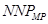= GDP – Depreciation

= 500 – 50

= Rs.450

(iii)= NNP – Sales tax

= 450 – 30

= Rs.420

NCERT Solutions class 12 Economics National income accounting

(iv) PI =– Retained earnings

= 420 – 220

= Rs.200

(v) PDI = PI – Income tax

= 200 – 20

= Rs.180

### 11.The value of the nominal GNP of an economy was Rs 2,500 crores in a particular year. The value of GNP of that country during the same year, evaluated at the prices of same base year, was Rs 3,000 crores. Calculate the value of the GNP deflator of the year in percentage terms. Has the price level risen between the base year and the year under consideration?

Ans: Nominal GNP = Rs.2500

Real GNP = Rs.3000

GNP deflator=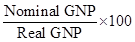So,

GNP deflator =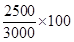= 83.33%

No, the price level has fallen down by 16.67 % [(100 – 83.33) %].

### 12. Write down some of the limitations of using GDP as an index of welfare of a country.

Ans: Limitations of using GDP as an indicator are as follows:

(i) Non-monetary exchanges: GDP measures the goods and services produced in an economy during a particular period of time. However, it does not take into account those transactions that do not come under monetary terms. In less developed countries there are non-monetary exchanges, particularly in rural areas. Hence, these transactions remain outside the domain of GDP. The household sector and volunteer sectors get ignored in GDP.

(ii) Inflation: GDP does not take into account the level of prices in a country. Because of inflation, the cost of living increases leading to a decrease in the standard of living. The loss of welfare due to this decrease is not taken into consideration by GDP as an index of welfare.

(iii) Externalities: Increase in the national income is associated with increased levels of pollution, accidents, disasters, shortage and depletion of natural resources, etc. These factors affect human health and lead to ecological degradation. GDP fails to consider the costs or valuations of such factors.

(iv) Income pattern: GDP disregards the income distribution pattern. The increase in aggregate national income may be a result of the increase in income of a few individuals. Thus, this may lead to false interpretation of social welfare.

(v) Welfare: GDP ignores the welfare component as the goods and services produced may or may not add to the welfare to a society. For example, the production of goods, like guns, narcotic drugs, high-end luxurious goods increase the monetary value of production, but they do not add to the welfare of the majority of population.

## NCERT Solutions for Class 12 Economics

NCERT Solutions class 12 Economics National income accounting PDF (Download) Free from myCBSEguide app and myCBSEguide website. Ncert solution class 12 Economics includes text book solutions from both part 1 and part 2. NCERT Solutions for CBSE Class 12 Economics have total 12 chapters. 12 Economics NCERT Solutions in PDF for free Download on our website. Ncert Economics class 12 solutions PDF and Economics ncert class 12 PDF solutions with latest modifications and as per the latest CBSE syllabus are only available in myCBSEguide

## CBSE App for Students

To download NCERT Solutions for class 12 Physics, Chemistry, Biology, History, Political Science, Economics, Geography, Computer Science, Home Science, Accountancy, Business Studies and Home Science; do check myCBSEguide app or website. myCBSEguide provides sample papers with solution, test papers for chapter-wise practice, NCERT solutions, NCERT Exemplar solutions, quick revision notes for ready reference, CBSE guess papers and CBSE important question papers. Sample Paper all are made available through the best app for CBSE students and myCBSEguide website.